# Spectrum Analyzer

Spectrum analysis primarily measures power, frequency, and noise. It is concerned primarily with characterizing signal components (such as its spurious and harmonic components, modulation, noise, etc.) Spectrum analysis locates frequencies where microwave energy exists.

The following stuff on spectrum analyzer measurements is mostly taken from a 25 year-old Hewlett Packard 8565A spectrum analyzer manual (with some "polishing" by the Unknown but All-Knowing Editor), with permission from Agilent (thanks, Sylvia!) who would appreciate it if you bought \$100K worth of new test equipment after being inspired by reading this material. Or you can check out the options available on eBay!

Your own spectrum analyzer may be a different model, but the techniques described below are still valid. You can score big points with the Pointy-Haired Boss by making measurements up to 22 GHz with that 112 pound boat anchor of an analyzer that has been taking up shelf space in your lab, while your whiney lab mates cry about overhead cuts that have put off the purchase of a newer model.

Distortion measurements

Distortion in amplifiers

Third order intercept measurement

Distortion in mixers

Distortion in oscillators

Modulation measurements

Amplitude modulation (AM) measurement

Frequency modulation (FM) measurement

Pulsed RF measurements

Noise measurements

Noise figure (NF) measurement

Electromagnetic interference (EMI) measurement

## Distortion measurements

Distortion measurement is an area where the spectrum analyzer makes a significant contribution. There are two basic types of distortion that are usually specified by the manufacturer: harmonic distortion and two-tone, third-order intermodulation distortion. The third-order intermodulation products are represented by: 2f1 - f2 and 2f2 - f1, where f1 and f2 are the two-tone input signals.

The HP 8565A can measure harmonic distortion products up to 100 dB down in the 1.7 to 22 GHz frequency range. Third-order intermodulation products can also be measured up to 100 dB down, depending on signal separation and frequency range. In all, the HP 8565A is capable of making a wide variety of distortion measurements with speed and precision.

### Distortion in amplifiers

All amplifiers generate some distortion at the output and these distortion products can be significant if the amplifier is overdriven with a high-level input signal. The test setup in Figure 1 was used to measure the third-order intermodulation products of a microwave FET (field-effect transistor) amplifier. Directional couplers and attenuators were used to provide isolation between sources.

Figure 2 is a CRT photo of a two-tone, third-order intermodulation measurement. The third-order products P(2f1 - f2) and P(2f2 - f1) are 50 dB below the two-tone signals P(f1) and P(f2). The difference between the power levels of the two-tone signals and the intermodulation products is known as the "intermodulation ratio". Note that if you adjust the power levels of the two tones P(f1) and P(f2) to be exactly equal in power, the power levels of the intermodulation products will be exactly equal as well. So tweak the power levels of P(f1) and P(f2) carefully or you will have several choices on the display to calculate the intermodulation ratio from.

### Third-order intercept point measurement

If you measured and plotted the power levels P(f1) and P(2f2-f1) versus input power, eventually at some power level they would be equal. (We promise to get into this more in-depth in a future chapter on receivers. Unknown Editor) This is known as the "third-order intercept point", sometimes abbreviated TOI, sometimes IP3 or even other weird ways. The higher the TOI, the more power an amplifier can handle.

The beautiful thing about the scope output in Figure 2 is that from this one picture the amplifier's TOI can be calculated. How is this possible without taking multiple sets of data and plotting the relationship between P(f1) and P(2f2-f1)? A phenomenon you need to be aware of is that the third-order modulation products increase 3 dB for every 1 dB that the input power of the two tones are increased, while the two-tone power levels only increase 1 dB/dB (thanks for the correction, Barrett!) Why does this happen? Let some scientist worry about that, just be glad that it does and use it to calculate the TOI from the data in Figure 2:

P(f1) = 0 dBm

P(2f2-f1) = -50 dBm

Intermodulation Ratio = P(f1) - P(2f2-f1) = 0 dB - (-50 dB) = 50 dB

TOI = P(f1) +1/2(Intermodulation Ratio) = 0 dBm + 25 dB = 25 dBm

How close should the two frequencies be for measuring intermodulation ratio and TOI? Why argue with an HP manual? Use 100 MHz separation, with 1 MHz resolution bandwidth.

One word of caution about TOI measurements: the actual intercept point is only a mathematical construct; you should never try to measure it directly. Chances are the DUT will blow up well before the four output tones are all equal in power!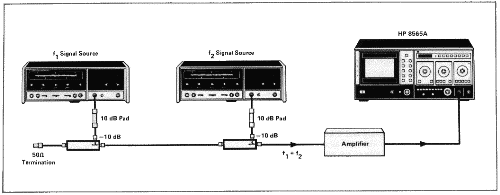Figure 1. Two-tone test setup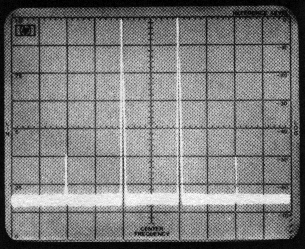RES BW 1 MHz, REF LEVEL 0 dBm, LOG SCALE 10 db/
FREQUENCY 5.950 GHz, FREQ SPAN/DIV 50 MHz

Figure 2. Two-tone, third-order intermodulation products

### Distortion in mixers

Mixers utilize the non-linear characteristics of an active or passive device to achieve a desired frequency conversion. This results in some distortion at the output due to the inherent non-linearity of the device. Figure 3 illustrates the test setup and CRT photograph of a typical mixer measurement.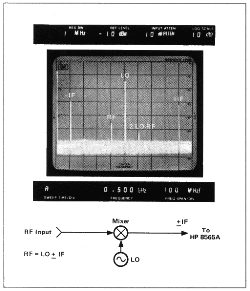Figure 3. Mixer measurement

From a single display, the following information was determined:

Conversion loss (SSB):
RFin - IF = (-30) - (-40)= 10 dB

LO to IF Isolation:
LOin - LOout(IF) = (+5) - (-25)=30 dB

RF to IF Isolation:
RFin - RFout(IF) = (-30) - (-57)=27 dB

Third-Order Distortion Product (2 LO - RF)= -64 dBm @ 600 MHz.

### Distortion in oscillators

Distortion in oscillators may be harmonically or non-harmonically related to the fundamental frequency. Non-harmonic oscillator outputs are usually termed spurious. Both harmonic and spurious outputs of an oscillator can be minimized with proper biasing and filtering techniques. The HP 8565A can monitor changes in distortion levels while modifications to the oscillator are made. In the full-hand modes, a tuning marker can be located under any signal response to determine its frequency and hence its relationship to the oscillator's fundamental frequency. Figure 4 is a CRT photo of the fundamental and second harmonic of an S-band (2-4 GHz) YIG oscillator. The internal preselector of the HP 8565A enables the analyzer to measure a low-level harmonic in the presence of a high level fundamental. The photo was obtained by adjusting the PERSIST control to allow storage of the trace and then tuning the oscillator over a narrow band.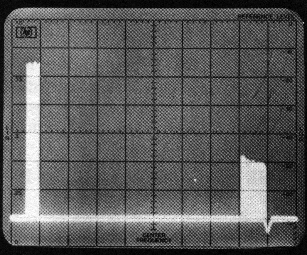RES BW 3 MHz, REF LEVEL 0 dBm, LOG SCALE 10 dB/
FREQUENCY 3.864 GHz, FREQ SPAN/DIV F Hz

Figure 4. Oscillator fundamental and second harmonic

## Modulation measurements

### Amplitude modulation (AM) measurement

The wide dynamic range of the spectrum analyzer allows accurate measurement of modulation levels. A 0.06% modulation is a logarithmic ratio of 70 dB, which is easily measured with the HP 8565A. Figure 5 is a signal with 2% AM; a log ratio of 40 dB.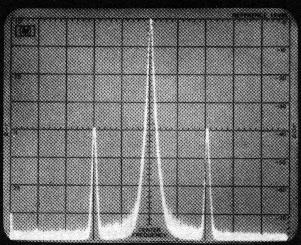RES BW 3 kHz, REF LEVEL -20 dBm, LOG SCALE 10 dB/
FREQUENCY 1.067 GHz, FREQ SPAN/DIV 50 kHz

Figure 5. 2% Amplitude Modulation

When the analyzer is used as a manually-tuned receiver (ZERO SPAN), the AM is demodulated and viewed in the time domain. To demodulate an AM signal, uncouple the RESOLUTION BW and set it to a value at least twice the modulation frequency. Then set the AMPLITUDE SCALE to LIN and center the signal, horizontally and vertically, on the CRT (see Figure 6). By pushing ZERO SPAN and VIDEO triggering, the modulation will be displayed in the time domain (see Figure 7.) The time variation of the modulation signal can then be measured with the calibrated SWEEP TIME/DIV control.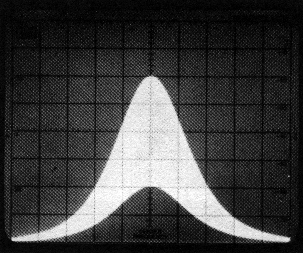RES BW 1 MHz, REF LEVEL -18 dBm, LOG SCALE 10 dB/
FREQUENCY 1.067 GHz, FREQ SPAN/DIV 50 kHz

Figure 6. Linear Amplitude Display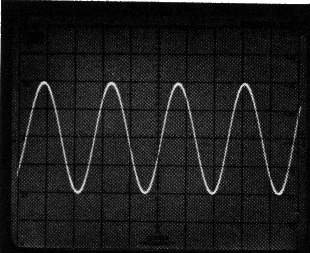RES BW 1 MHz, REF LEVEL -18 dBm, LOG SCALE 10 dB/
FREQUENCY 0.550 GHz, FREQ SPAN/DIV 0 kHz

Figure 7. Demodulated AM signal in ZERO SPAN

### Frequency modulation (FM) measurement

For frequency modulated signals, parameters such as modulation frequency (fm), modulation index (m), peak frequency deviation of carrier (Df peak) are all easily measured with the HP 8565A. The FM signal in Figure 8 was adjusted for the carrier null which corresponds to m = 2.4 on the Bessel function. The modulation frequency, fm, is simply the frequency separation of the sidebands which is 50 kHz. From this, we can calculate the peak frequency deviation of the carrier (Df peak) with the following equation: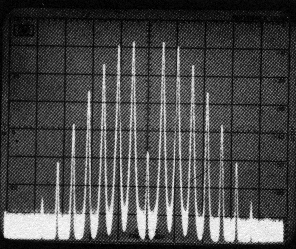RES BW 3 kHz, REF LEVEL -22dBm, LOG SCALE 10 dB/
FREQUENCY 0.098 GHz, FREQ SPAN/DIV 100 kHz

Figure 8. FM signal

m=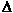f peak/fm orf peak 2.4 x 50 kHz 120 kHz

Although the HP 8565A does not have a built-in discriminator, FM signals can be demodulated by slope detection. Rather than tuning the signal to the center of the CRT as in AM, the slope of the IF filter is tuned to the center of the CRT instead. At the slope of the IF filter, the frequency variation is converted to amplitude variation. When ZERO SPAN is selected, the amplitude variation is detected by the analyzer and displayed in the time domain as shown in Figure 9. In FM, the RESOLUTION BW must be increased to yield a display similar to Figure 10 before switching to ZERO SPAN.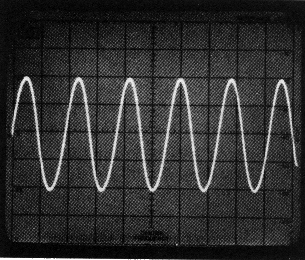RES BW 300 kHz, REF LEVEL -23 dBm,
FREQUENCY 0.098 GHz, FREQ SPAN/DIV 0 kHz

Figure 9. Demodulated FM signal in ZERO SPAN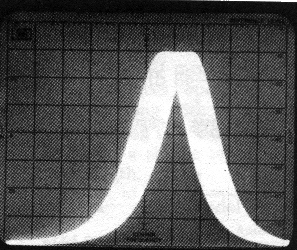RES BW 300 kHz, REF LEVEL -23 dBm,
FREQUENCY 0.098 GHz, FREQ SPAN/DIV 200 kHz

Figure 10. Slope detection of FM signal

## Pulsed RF measurements

A pulsed RF signal is a train of RF pulses having constant amplitude. If you haven't encountered a pulsed RF signal in the lab, you ain't done squat! Some pulsed RF signal parameters parameters that can be determined directly on a spectrum analyzer include PRF (pulse repetition frequency), PRI (pulse repetition interval, which is the reciprocal of PRF), pulse width, duty cycle, peak and average pulsed power, and the on/off ratio of the modulator. Figure 11 illustrates a line spectrum presentation of a pulsed RF signal.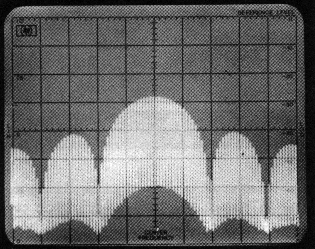RES BW 10 kHz, REF LEVEL -20 dBm, LOG SCALE 10 dB/
FREQUENCY 2.402 GHz, FREQ SPAN/DIV 500 kHz

Figure 11. Line spectrum of pulsed RF signal

A line spectrum (as opposed to a pulsed spectrum) is an actual Fourier representation of a pulsed RF signal in the frequency domain; all the spectral components of the signal are fully resolved. To obtain a line spectrum on the analyzer, the "rule of thumb" to follow is that the RESOLUTION BW be less than 0.3 x PRF. This ensures that individual spectral lines will be resolved. From the line spectrum shown in Figure 11, it is possible to measure the following parameters:

PRF = 50 kHz (the spacing between the spectral lines)

PRI = 1/PRF = 20 microseconds

lobe width = 1MHz

mainlobe power = -48 dBm

Then from the above measurement the following data can be calculated:

Pulse width 1/(lobe width) = 1/1MHz=1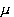s

Duty Cycle=PRF/(lobe width) = 50kHz/(1MHz) = 0.05

A factor to consider when measuring the amplitude of a pulsed RF signal is the pulse desensitization factor. The mainlobe power of a pulsed RF signal does not represent the actual peak power of the signal. This is because a pulsed signal has its power distributed over a number of spectral components and each component represents a fraction of the peak pulse power. The spectrum analyzer measures the absolute power of each spectral component. To determine the peak pulse power in a line spectrum, a pulse desensitization factor (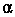L) must be added to the measured mainlobe power. The desensitization factor is a function of the duty cycle and is represented by the following equation:L = 20 log (duty cycle)

For a duty cycle of 0.05,L = -26 dB. Hence the peak pulse power in Figure 12 is -22 dBm (-48 dBm +26 dB).

An alternate method of measuring a pulsed RF signal is in the pulse spectrum mode. In a pulse spectrum, the individual spectral lines are not resolved. If the RESOLUTION BW of the analyzer is greater than 1.7 x PRF, then the pulsed RF signal is being viewed in the pulse spectrum. Using the pulse spectrum enables a wider resolution bandwidth to be used. Two benefits result from this: first, the signal-noise ratio is increased because the pulse amplitude increases linearly with the resolution bandwidth (RBW) whereas random noise increases only proportionally to the square-root of the video bandwidth (VBW)1/2. Hence the signal-noise ratio of the analyzer is effectively increased. Secondly, faster sweep times can be used because of the wider resolution bandwidths. The HP 8565A has a 3 MHz RESOLUTION BW which enables it to effectively display pulsed RF signals in the pulse spectrum. The 3 MHz bandwidth, along with fast sweep times, also enables narrow pulse widths to be measured in the time domain. Figure 12 illustrates a signal in the pulse spectrum. The same signal is demodulated with the analyzer in Figure 13.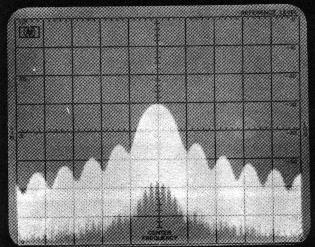RES BW 30 kHz, REF LEVEL -1 dBm, LOG SCALE 10 dB/
FREQUENCY 2.402 GHz, FREQ SPAN/DIV 100 kHz

Figure 12. Pulse spectrum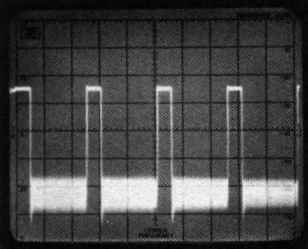RES BW 3 MHz, REF LEVEL 0 dBm, LOG SCALE 10 dB/
FREQUENCY 2.402 GHz, FREQ SPAN/DIV 0 MHz

Figure 13. Demodulated pulsed RF signal in ZERO SPAN

An additional factor to consider when measuring pulsed RF signals is the VIDEO FILTER control. In general, the VIDEO FILTER should be left in the OFF position when measuring pulsed RF signals. Adding video filtering will desensitize a pulsed signal and limit its displayed amplitude. Hence, when monitoring pulsed signals in a fullband mode, it is important to use the F mode rather than the FULL BAND pushbutton mode. The FULL BAND mode automatically engages a 9 kHz VIDEO FILTER (0.003 x 3 MHz) which will limit the displayed amplitude of the pulse.

## Noise measurements

Applications involving noise measurements include oscillator noise (spectral purity), signal-noise ratio, and noise figure. The NOISE AVG position of the VIDEO FILTER control can be used to measure the analyzer sensitivity or noise power from 0.01 to 22 GHz.

### Noise figure measurement

(Note: the spectrum analyzer should only be used for noise figure measurements by poor bastards that don't have a noise figure meter available. Unknown Editor.) The test setup in Figure 14 is used to make a swept noise figure measurement of an amplifier. In general, this measurement involves determining the total gain of the amplifier under test and the pre-amp. Then the input of the amplifier is terminated and its noise power is measured. The noise figure of the amplifier will then be the theoretical noise power (KTB) minus the total gain and the amplifier noise power. Figure 15 is a photo of an amplifier's noise power output from 0.012 to 1.3 GHz.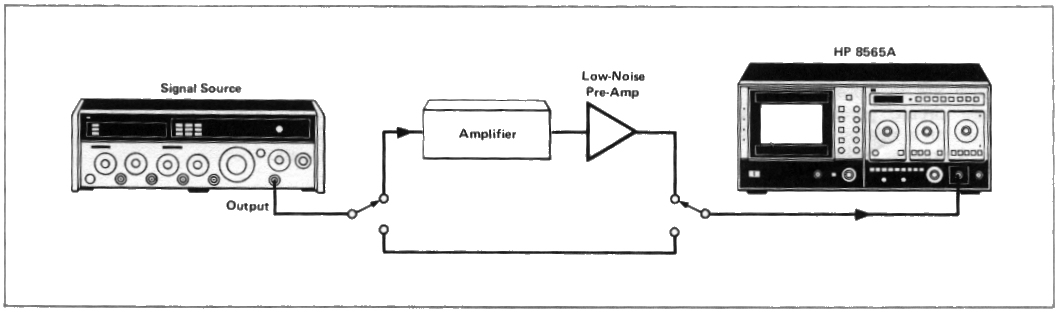Figure 14. Swept noise figure test setup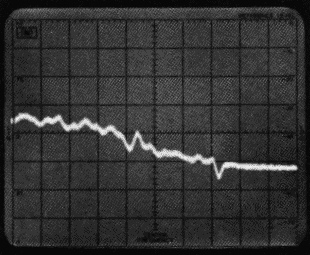RES BW 3 MHz, REF LEVEL -30 dBm, LOG SCALE 10 dB/
FREQUENCY 1.300 GHz, FREQ SPAN/DIV F Hz

Figure 15. Noise power measurement

## Electromagnetic interference (EMI) measurement

The overall objective of EMI measurement is to assure compatibility between devices operating in the same vicinity. The HP 8565A, along with an appropriate transducer, is capable of measuring either conducted or radiated EMI. Figure 16 illustrates a simple setup used for measuring radiated field strength.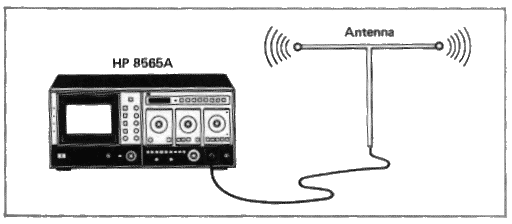Figure 16. Field strength test setup

The antenna is used to convert the radiated field to a voltage for the analyzer to measure. The field strength will be the analyzer reading plus the antenna correction factor. Figure 17 illustrates a typical signal generated by a DUT (Device Under Test) with spurious radiation.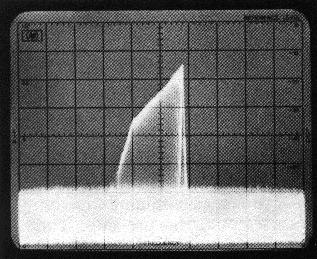RES BW 300 kHz, REF LEVEL -10 dBm, LOG SCALE 10 dB/
FREQUENCY 0.226 GHz, FREQ SPAN/DIV 10 MHz

Compatibility is also important for high-frequency circuits which are in close proximity to each other. In a multistage circuit, parasitic oscillation from one stage can couple to it nearby stage and cause unpredictable behavior. A popular technique used to search for spurious radiation is with an inductive loop probe. The loop probe is simply a few turns of wire that attaches to the spectrum analyzer with a flexible coaxial cable. (See Figure 18.)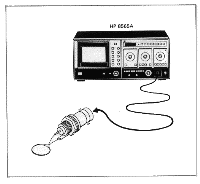Figure 18. Loop probe

Various parts of the circuit can then be "probed" to identify the location as well as the frequency and relative amplitude of a spurious signal. Once the spurious signal has been identified, design techniques can be implemented to reduce or eliminate the cause of interference.

Author : Unknown Editor